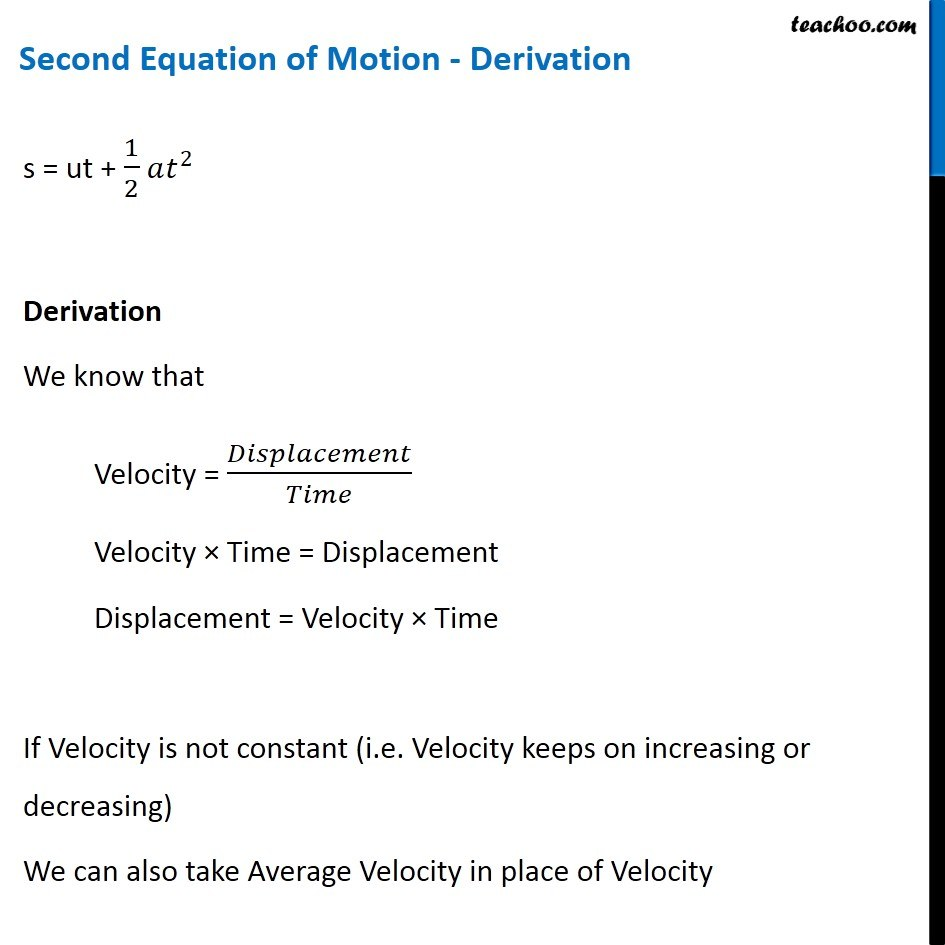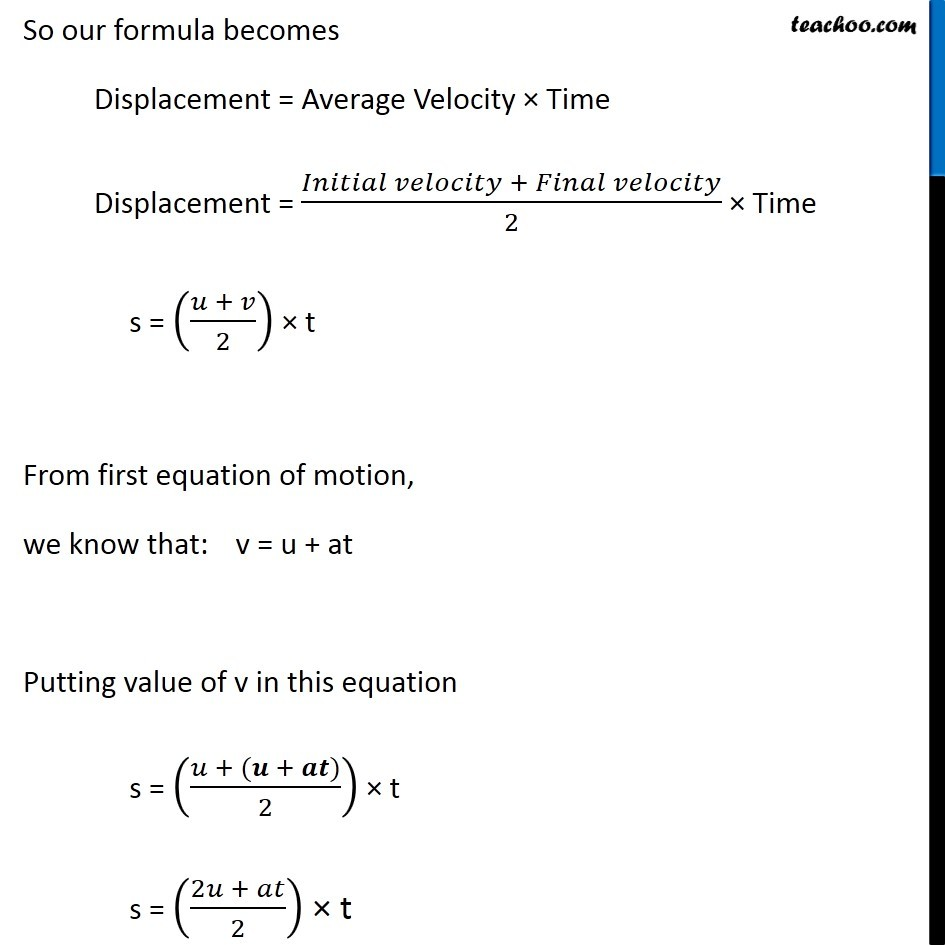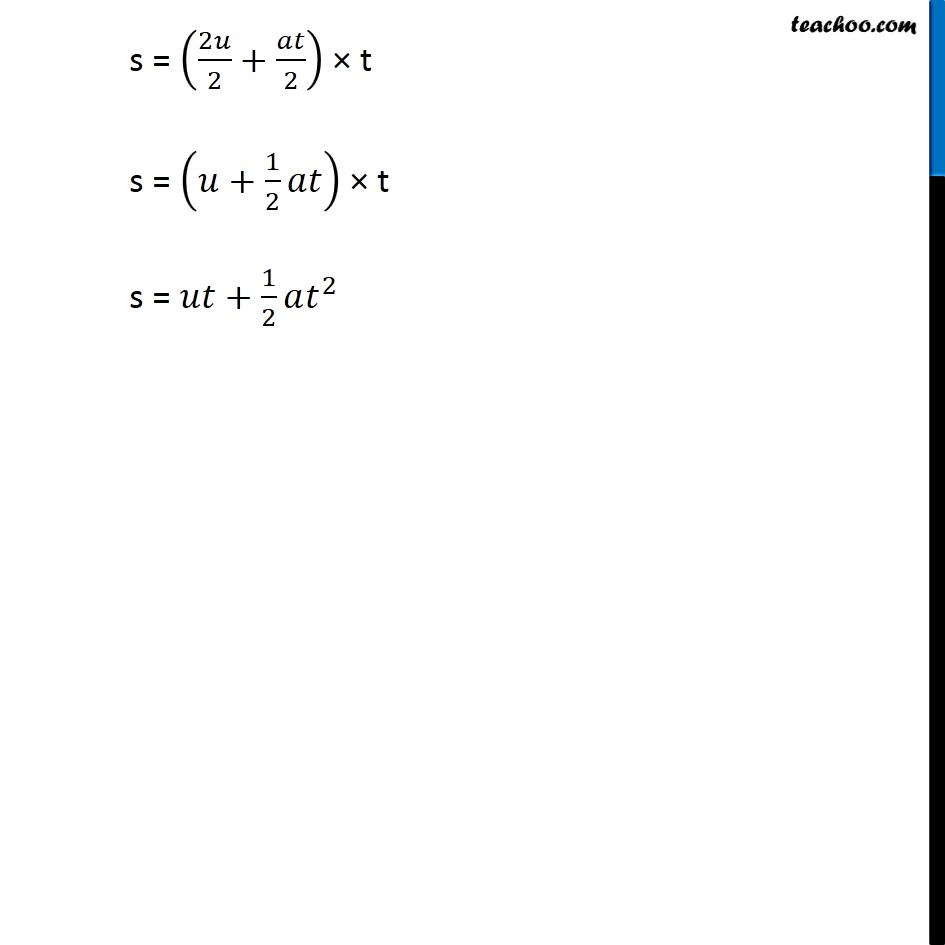Concepts

Class 9
Chapter 8 Class 9 - Motion

it is denoted by

s = ut + ½ at 2

Distance=Initial Velocity × Time + 1/2acceleration × time 2

Where

s = Distance Travelled

u = Initial Velocity

t = time taken

a = acceleration

#### How is this Equation Derived?## Questions

Q 1 Page 109 - A bus starting from rest moves with a uniform acceleration of 0.1 m s-2 for 2 minutes. Find (a) the speed acquired, (b) the distance travelled

Q 4 Page 110 - A racing car has a uniform acceleration of 4 m s-2. What distance will it cover in 10 s after start?

NCERT Question 4 - A motorboat starting from rest on a lake accelerates in a straight line at a constant rate of 3.0 m s–2 for 8.0 s. How far does the boat travel during this time?

Example 8.6 - A car accelerates uniformly from 18 km h–1 to 36 km h – 1 in 5 s.Calculate (i) the acceleration and (ii) the distance covered by the car in that time.

Example 8.7 - The brakes applied to a car produce an acceleration of 6 m s-2 in the opposite direction to the motion. If the car takes 2 s to stop after the application of brakes, calculate the distance it travels during this time.

Get live Maths 1-on-1 Classs - Class 6 to 12

### Transcript

Second Equation of Motion - Derivation s = ut + 1/2 𝑎𝑡^2 Derivation 𝜋× We know that Velocity = 𝐷𝑖𝑠𝑝𝑙𝑎𝑐𝑒𝑚𝑒𝑛𝑡/𝑇𝑖𝑚𝑒 Velocity × Time = Displacement Displacement = Velocity × Time If Velocity is not constant (i.e. Velocity keeps on increasing or decreasing) We can also take Average Velocity in place of Velocity So our formula becomes Displacement = Average Velocity × Time Displacement = (𝐼𝑛𝑖𝑡𝑖𝑎𝑙 𝑣𝑒𝑙𝑜𝑐𝑖𝑡𝑦 + 𝐹𝑖𝑛𝑎𝑙 𝑣𝑒𝑙𝑜𝑐𝑖𝑡𝑦)/2 × Time s = ((𝑢 + 𝑣)/2) × t From first equation of motion, we know that: v = u + at Putting value of v in this equation s = ((𝑢 + (𝒖 + 𝒂𝒕))/2) × t s = ((2𝑢 + 𝑎𝑡)/2) × t s = (2𝑢/2+𝑎𝑡/2) × t s = (𝑢+1/2 𝑎𝑡) × t s = 𝑢𝑡+1/2 𝑎𝑡^2